Next: Butterworth-filter finding program Up: Spectrum and phase Previous: Relation of amplitude to

# A BUTTERWORTH-FILTER COOKBOOK

An ideal bandpass filter passes some range of frequencies without distortion and suppresses all other frequencies. Further thought shows that what we think of as the ideal bandpass filter, a rectangle function of frequency, is instead far from ideal, because its time-domain representationis noncausal and decays much too slowly with time for many practical uses. The appropriate bandpass filter is one whose time decay can be chosen to be reasonable (in combination with a reasonable necessary compromise on the shape of the rectangle). Butterworth filters fulfill these needs.

They are causal and of various orders, the lowest order being best (shortest) in the time domain, and the higher orders being better in the frequency domain. Well-engineered projects often include Butterworth filters. Unfortunately they are less often used in experimental work because of a complicated setting-up issue that I am going to solve for you here. I will give some examples and discuss pitfalls as well.

The main problem is that there is no simple mathematical expression for the filter coefficients as a function of order and cutoff frequency.

Analysis starts from an equation that for large-order n is the equation of a box:(21)
When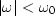, this Butterworth low-pass spectrum is about unity. When, the spectrum drops rapidly to zero. The magnitude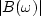(with some truncation effects to be described later) is plotted in Figure 14 for various values of n.butf
Figure 14
Spectra of Butterworth filters of various-order n.

Conceptually, the easiest form of Butterworth filtering is to take data to the frequency domain and multiply by equation (21), where you have selected some value of n to compromise between the demands of the frequency domain (sharp cutoff) and the time domain (rapid decay). Of course, the time-domain representation of equation (21) is noncausal. If you prefer a causal filter, you could take the Butterworth spectrum into a spectral-factorization program such as kolmogoroff().

The time-domain response of the Butterworth filter is infinitely long, although a Butterworth filter of degree n can be well approximated by a ratio of-order polynomials. Since, as we will see, n is typically in the range 2-5, time-domain filtering is quicker than FT. To proceed, we need to expressin terms of Z, where.This is done in an approximate way that is valid for frequencies far from the Nyquist frequency. Intuitively we know that time differentiation is implied by.We saw that in sampled time, differentiation is generally represented by the bilinear transform, equation ():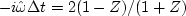.Thus a sampled-time representation of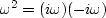is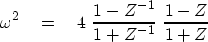(22)
Substituting equation (22) into (21) we find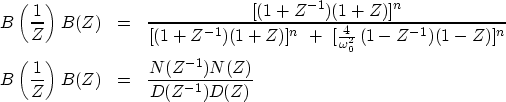(23) (24)
where the desired, causal, Butterworth, discrete-domain filter is B(Z)=N(Z)/D(Z). You will be able to appreciate the enormity of the task represented by these equations when you realize that the denominator in (23) must be factored into the product of a function of Z times the same function of Z-1 to get equation (24). Since the function is positive, it can be considered to be a spectrum, and factorization must be possible.Next: Butterworth-filter finding program Up: Spectrum and phase Previous: Relation of amplitude to
Stanford Exploration Project
10/21/1998